• 极差归一化

千次阅读 2017-08-29 16:42:07
极差归一化

极差归一化
目的：①消除计量单位差异，将评价指标的绝对值，转化为相对值。
②评价指标分为：正指标、逆指标和适度性指标。正指标是指标值越离，评价对象越优；逆指标是指标值越低，评价对象越差；而适度性指标是指标数越接近某一值，评价对象越优。
对于正指标，计算公式如下：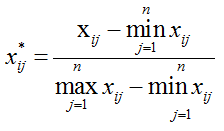对于逆指标，计算公式如下：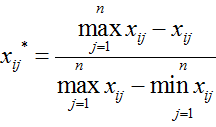对于适度指标，计算公式如下（其中Xi*为该指标最优值）：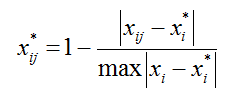matlab实现：

function [ X_out ] = rscore( X_in,N,x )
%RSCORE 此函数用来实现极差归一化
%函数实现按列归一化
%X_out:归一化后的矩阵
%X_in:矩阵，需要归一化的矩阵
%N: 正整数，N=1,正指标归一化，N = 2，逆指标归一化，N = 3，适度指标归一化
%x：行向量，N = 3时需要给出x，x为每一列数据的最优指标
n0 = size(X_in);
m = zeros(n0(1),1);
maxx = max(X_in);
minn = min(X_in);
m = maxx-minn;
X_out = zeros(n0);
if N == 1
for j = 1:n0(2)
for i = 1:n0(1)
X_out(i,j) = (X_in(i,j) - minn(j))/m(j);
end
end
elseif N == 2
for j = 1:n0(2)
for i = 1:n0(1)
X_out(i,j) = (maxx(j)-X_in(i,j))/m(j);
end
end
elseif N == 3
for k = 1:n0(2)
m(k) = max(abs(X_in(:,k)-x(k)));
end
for j = 1:n0(2)
for i = 1:n0(1)
X_out(i,j) = 1-abs(X_in(i,j)-x(j))/m(j);
end
end
end
end

展开全文matlab
• 2016-6-10 17:12:12 上传下载附件 (15.05 KB)这是怎么回事这是怎么回事2..不输入rscore函数的源代码，直接使用，会出现[R,xmin,xrang]=rscore(x)错误使用 rscoreNot enough input arguments.3.MATLAB 文件帮助: r...2016-6-10 17:12:12 上传

下载附件 (15.05 KB)

这是怎么回事

这是怎么回事

2..不输入rscore函数的源代码，直接使用，会出现

[R,xmin,xrang]=rscore(x)

错误使用 rscore

Not enough input arguments.

3.MATLAB 文件帮助: rscore，是这样写的。

The core function in Reed-Solomon decode.

MSG = rscore(CODE, K, TP, M, POW_M, T2) decodes a single codeword

vector CODE using the Reed-Solomon decoding technique. The message length

is K. The complete (and correct) list of all members in GF(2^M) is

in TP. The code word length is provided in POW_M, which equals

2^M - 1. The decoding result is provided in the output variable MSG.

[MSG, ERR] = rscore(CODE, K, TP, M, POW_M, T2) outputs the error

detected in the decoding.

[MSG, ERR, CCODE] = rscore(CODE, K, TP, M, POW_M, T2) outputs the

corrected codeword in CCODE.

NOTE: Unlike all of the other encoding/decoding functions,

this function takes exponential input instead of regular input for

processing. For example [-Inf, 0, 1, 2, ...] represents

[0 1 alpha, alpha^2, ...] in GF(2^m). There are 2^M elements in

GF(2^M). Hence, the input CODE represents 2^M * (2^M - 1) bits of

information. The decoded MSG represents 2^M * K bits of information.

To speed computation, no error-checking is placed in this function,

all input variables must be present.

没有看明白。

展开全文• 解答：当数据(x)按照最小值中心化后，再按极差(最大值-最小值)缩放，数据移动了最小值个单位，并且会被收敛到[0，1]之间，而这个过程，就称作数据归一化(Normalization，又称Min-Max Scaling)。在sklearn当中，我们...

问题一：什么是归一化？

解答：

当数据(x)按照最小值中心化后，再按极差(最大值-最小值)缩放，数据移动了最小值个单位，并且会被收敛到[0，1]之间，而这个过程，就称作数据归一化(Normalization，又称Min-Max Scaling)。在sklearn当中，我们使用preprocessing.MinMaxScaler来实现这个功能。

MinMaxScaler有一个重要参数，feature_range，控制我们希望把数据压缩到的范围，默认是[0，1]。

问题二：怎样实现归一化？

代码：

# 先切分整体的数据集， 利用mms，学习训练集的信息， 用他们来转换训练集，转换测试集

# 可以避免之前所描述的信息的泄露

X_train, X_test, y_train, y_test = train_test_split(X, # array或者是DF

y, # 标签

test_size=0.3, # 切分出来的测试集的占比

random_state=210# 随机数种子

)

# 实现归一化

from sklearn.preprocessing import MinMaxScaler, StandardScaler

# 实例化

mms = MinMaxScaler()

mms = mms.fit(X_train)

X_train_ = mms.transform(X_train)

X_test_ = mms.transform(X_test)

# 训练和导出结果一步达成

clf = KNeighborsClassifier(n_neighbors = 4)

clf.fit(X_train_, y_train)

clf.score(X_train_, y_train), clf.score(X_test_, y_test)

展开全文• 继续之前的数据和工作，进行数据的标准处理，具体数据可从下方博客链接获取 剔除异常值 ...这里采用极差变换： 放代码： import numpy as np import pandas as pd path = "E:/Mo...

继续之前的数据和工作，进行数据的标准化处理，具体数据可从下方博客链接获取

剔除异常值

放下表一和表二Excel的截图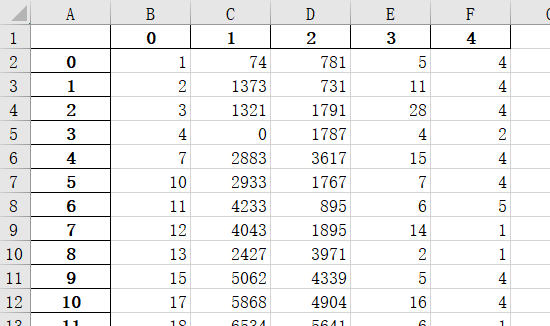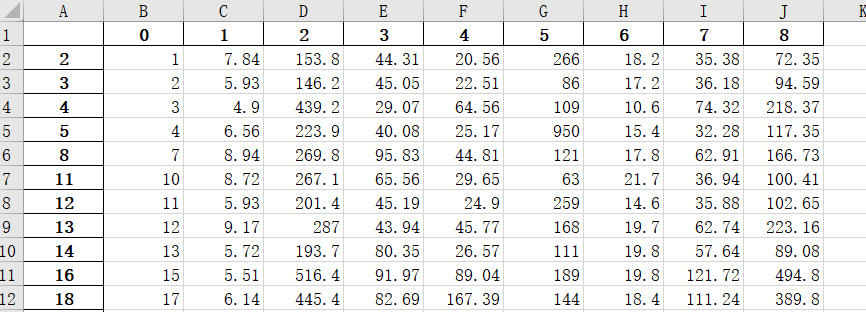大体思路：

实际问题中，不同数据可能有不同的性质和量纲，为进行模糊聚类，需进行标准化处理。这里采用极差变换：

放代码：

import numpy as np
import pandas as pd
path = "E:/Model_building/A/"
s1 = pd.DataFrame(s1.values)             # dataframe
s2 = pd.DataFrame(s2.values)
s1 = s1.drop([s1.columns], axis=1)    # 去掉0列
s2 = s2.drop([s2.columns], axis=1)

s1.columns = ['a', 'b', 'c', 'd', 'e']         # 之前作死删掉了列名，感觉还是用列名更方便
s2.columns = ['1', '2', '3', '4', '5', '6', '7', '8', '9']

s2['1'] = s2['1'].astype('int64')    # 输出发现表2的序号列为float，更改为int

看一下s1和s2的头部输出，其中a，1都为采样点的序号，b,c为xy坐标，d为海拔，e为地区类型，2到9为八种元素在每个采样点的浓度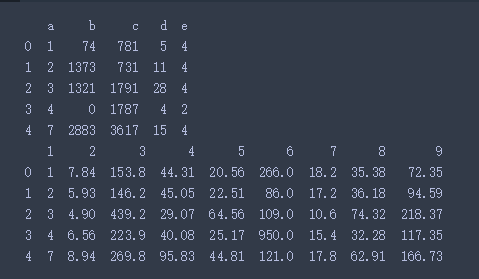s12 = pd.merge(s1, s2, how='inner', left_on='a', right_on='1')   # 将表1、2按照索引，合并为s12
del s12['1']

看一下s12的头部输出 ，这样就明了了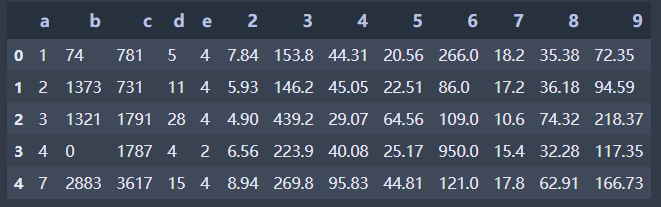path = "E:/Model_building/A/"
ma = s12.groupby(s12['e']).max().iloc[:, 4:12]
mi = s12.groupby(s12['e']).min().iloc[:, 4:12]     # 按照地区列分组，得出每种元素最大最小值
s122 = s12.set_index(['a','e'])    # 双重索引
print(mi,'\n')
s122.iloc[:, 3:12] = ( s122.iloc[:, 3:12] - mi )/(ma-mi)   # 极差变换
s122.to_excel(path+"sheet_standardized.xlsx")

在进行极差变换时，由于s122和mi相减时，由于s122每种e有多个，所以一直报错 ，如下：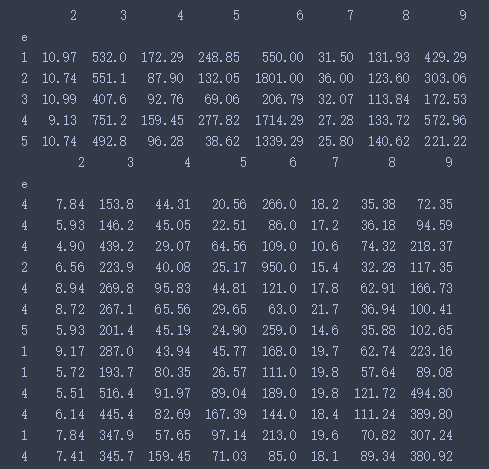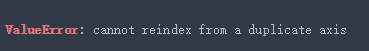不能有重复索引，所以设置一个双重索引（即地区和序号），这样在相减时，自动匹配对应的行（e）和列（元素）

pathx = "E:/Model_building/A/cumcm2011A附件_数据.xls"
p = s12.iloc[:,5:].groupby(s12['e']).mean()  # 每个地区每种元素的均值
print(p)
s122 = s122.reset_index()   # 取消索引，以便下一步引用‘e’
p1 = s122.groupby(s122['e']).mean().iloc[:, 5:]   # 每个地区每种元素，经过极差变换后的均值
print(p1)
p1.to_excel(path+"sheet1-6.xlsx")   # 保存
p.to_excel(path+"sheet1-7.xlsx")

放一下两个表的结果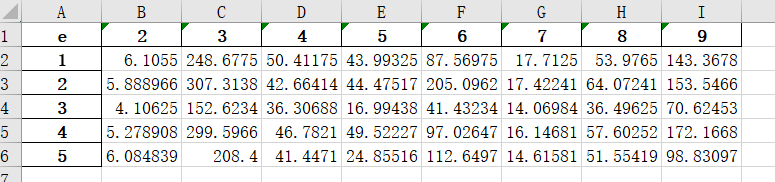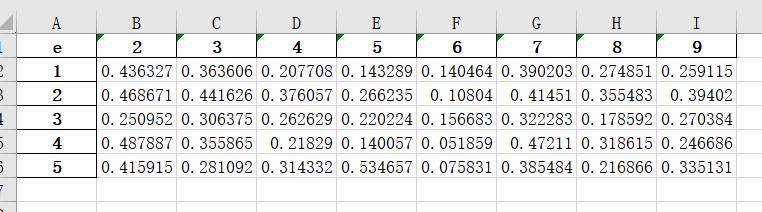展开全文Python 数据分析 数学建模
• /** * * 数据归一化 * */ public class GUIYi{ /** * @param args * @throws IOException */ public static void main(String[] args) throws IOException { File fileName = new File("C:\\Users\\Administrator\\...java 数据归一化
• 公众号后台回复“图书“，了解更多号主新书内容作者：小一来源：小一的学习笔记今天说一个比较重要的内容，无论是在算法建模还是在数据分析都比较常见：数据归一化和标准化。开始之前，请你先把网上看...算法 python 机器学习 人工智能 数据分析
• 数据归一化和代码实现

万次阅读 2018-05-15 17:41:47
数据标准化（归一化）处理是数据挖掘的一项基础工作，不同评价指标往往具有不同的量纲和量纲单位，这样的情况会影响到数据分析的结果，为了消除指标之间的量纲影响，需要进行数据标准化处理，以解决数据指标之间的...
• 在机器学习中，数据归一化是非常重要...线性变换和极差法（线性归一化） 将原始数据线性化的方法转换到[0 1]的范围，该方法实现对原始数据的等比例缩放。通过利用变量取值的最大值和最小值（或者最大值）将原始数据转换
• 首先归一化是为了后面数据处 理的方便,...关于神经网络(matlab)归一化的整理 关于神经网络归一化方法的整理 由于采集的各数据单位不一致,因而须对数据进行[-1,1]归一化处理,归一化 方法主要有如下几种,......在matl...
• 数据标准化（归一化）处理是数据挖掘的一项基础工作，不同评价指标往往具有不同的量纲和量纲单位，这样的情况会影响到数据分析的结果，为了消除指标之间的量纲影响，需要进行数据标准化处理，以解决数据指标之间的...
• 一、特征二值化二、特征归一化(一)、总和标准化(二)、标准差标准化(三)、极大值标准化(四)、极差标准化(区间放缩法，0-1标准化)三、连续特征变换四、定性特征哑编码：One-hot编码数据变换数据变换即对数据进行规范化...
• 示例原始数据 这部分计算量大，在excel上演练 欢迎指正！！数据分析
• 为何要归一化·提升模型的收敛速度·提升模型的精度·总结如何归一化和标准化min-max(0-1) 标准化z-score(标准) 标准化nonlinearity(非线性) 归一化 为何要归一化 ·提升模型的收敛速度 如下图，观察x1，x2的取值...归一化 深度学习
• 为什么要数据归一化Feature Scaling由于原始数据值的范围差异很大，因此在某些机器学习算法中，如果没有归一化，目标函数将无法正常工作。例如，许多分类器通过欧几里得距离来计算两点之间的距离。如果其中一个要素...
• 1，标准归一化。 　将原始数据集归一化为均值为0、方差1的数据集，归一化公式如下： x∗=x−μδ x^* = \frac{x-\mu}{\delta} 其中μ\mu为所有样本数据的均值，δ\delta为所有样本数据的标准。 2，最大最小归一...matlab 归一化
• 这里主要讲连续型特征归一化的常用方法。 连续型特征还有一种处理方式是，先分桶/分箱（如等频/等距的分）[待写]进行离散化后再使用离散数据的处理方法。 离散数据处理参考[数据预处理：独热编码（One-Hot ...数据标准化 归一化
• 而在第二种归一化方式中，新的数据由于对方进行了归一化，这时候每个维度的量纲其实已经等价了，每个维度都服从均值为0、方差1的正态分布，在计算距离的时候，每个维度都是去量纲化的，避免了不同量纲的选取对距离...机器学习 归一化
• 标准化和归一化介绍： （1）标准化 　标准化需要计算特征的均值和标准，公式表达为： 　使用preproccessing库的StandardScaler类对数据进行标准化的代码如下 from sklearn.preprocessing import StandardScaler ...python
• 请问在excel里可以直接做归一化处理吗归一化是一种计算的方式，即将纲的表达式，经换，化为纲的表达式，成为标量。 在多种计算中都经常用到这种方法。归一化是一种无量纲处理手段，使物理系统数值的绝对值变成某种...
• 2）极差变换法（离差标准）： 缺点：(1)当有新数据加入时，会导min(x)和max(x)的变化，需要重新定义。 (2)存在极端的最大值或者最小值。 适用于数据量较小的工程。 3）0均值标准（Z-score方法） 适用...
• 目录 数据变换的目的 数据变换的七种常见方式 初值化变换 均值化变换 百分比变换 倍数变换 归一化变换 极差最大值化变换 区间值化变换 1. matlab ...
• 一、归一化 对原始数据缩放到 0-1 之间，是线性变换。也叫最大最小标准化，离散标准化。 区间也可以设置为其他，一般为 0 - 1。 公式： X=x−minmax−min X = \frac{x-min}{max-min } X=max−minx−min​ min 为数据...sklearn 机器学习 python 归一化 标准化
• 1.极差变换法 2. 0均值标准化（Z-score方法） 1.Max-Min（线性归一化） Max-Min归一化是对原始数据进行线性变化，利用取值的最大值和最小值将原始数据转换为某一范围的数据 缺点：归一化过程与最大值和最小值有关...归一化 标准化方法 需要归一化的算法 应用场景
• 标准化与归一化1、标准化(Standardization)和归一化(Normalization)概念1.1、定义1.2、联系和差异一、联系二、差异1.3、标准化和归一化的多种方式2、标准化、归一化的原因、用途3、什么时候Standardization，什么...大数据 数据处理 标准化 归一化
• 数据归一化方法大全

千次阅读 2020-12-18 19:47:48
数据归一化方法大全在数据分析之前，我们通常需要先将数据标准化(normalization)，利用标准化后的数据进行数据分析。数据标准化也就是统计数据的指数化。数据标准化处理主要包括数据同趋化处理和无量纲化处理两个...正负数据如何归一化...